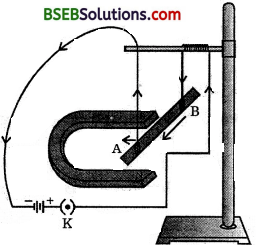# Bihar Board Class 10 Science Solutions Chapter 13 Magnetic Effects of Electric Current

## BSEB Bihar Board Class 10 Science Solutions Chapter 13 Magnetic Effects of Electric Current

Bihar Board Class 10 Science Solutions Chapter 13 Magnetic Effects of Electric Current Textbook Questions and Answers, Additional Important Questions, Notes

### Bihar Board Class 10 Science Chapter 13 Magnetic Effects of Electric Current InText Questions and Answers

Intext Question (Page 224)

Question 2.
Why does a compass needle get deflected when brought near a bamagnet ?
Compass needle is a tiny magnet, so, due to force of repulsion or attraction between the poles of a magnet, there is deflection in the compass needle.

Intext Questions (Page 228)

Question 1.
Draw magnetic field arotmd a bar magnet.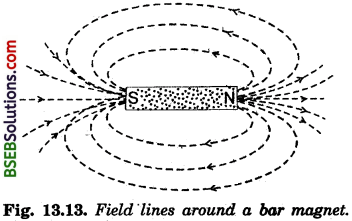Question 2.
List the properties of magnetic field lines.
The magnetic field lines have the following properties :
(a) These imaginary lines originate from the north pole and ; terminate at the south pole.
(b) These lines are parallel to each other and do not intersect.
(c) The direction of tangent at a point on a magnetic field ^ line indicates the direction of magnetic field at that point.
(d) At a point in the magnetic field, higher the number of field lines passing through that point, higher will be the value of the magnetic field.

Question 3.
Why don’t two magnetic lines of forces intersect each other ?
Two magnetic lines of force cannot intersect because we cannot have two directions of the magnetic field at the same point.

Intext Questions (Page 229)

Question 1.
Consider a circular loop of wire lying in the plane of the table. Let the current pass through the loop clockwise. Find out the direction of the magnetic field inside and outside the loop.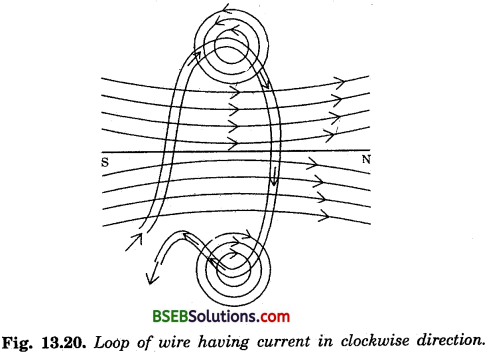Question 2.
The magnetic field in a given region is uniform. Draw a diagram to represent it.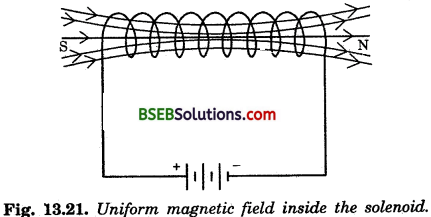Magnetic field lines are parallel straight lines inside the solenoid. So, the magnetic field is uniform inside the solenoid.

Question 3.
Choose the correct options :
The electric field inside a long straight solenoid carrying current :
(а) is zero.
(b) decreases as we move towards its end.
(c) increases as we move towards its end.
(d) is the same at all points.
(d) is the same at all points.

lntext Questions (Page 231)

Question 1.
Which of the following property of a proton can change while it improves freely in a magnetic field ? (There may.be more than one correct answer.)
(a) mass
(b) speed
(c) velocity
(d) momentum
(c) velocity
(d) momentum.Question 2.
In Activity 13.7, how do we think the’displacement of rod AB will be affected if the (i) current in rod AB is increased, (ii) a stronger horse shoe magnet is used and (iii) length of the rod AB is increased.
We know that when a current-carrying conductor is kept-in a magnetic field, the force acting on it is given by the expression :
F = B.I.L
Therefore,
(i) F α I.
As the value of current will increase in conductor, the value of force acting on it is increased. So there will be more displacement.
(ii) F α B.
As the value of B will increase, there will be more displacement in the rod AB.
(iii) F α L.
More the length of the rod AB, more will be the displacement in the rod.

Question 3.
The positively charged particle (a-particle) projected towards west is deflected towards the north by a magnetic field. The direction of magnetic field is :
(a) towards south
(b) towards east
(c) downward
(d) upward
(d) upward

Question 1.
State Fleming’s left-hand rule.
According to this rule, “Stretch the thumb, forefinger and middle finger of your left hand such that they are mutually perpendicular. If the first finger points in the direction of the magnetic field and the second finger in the direction of current, then the thumb will point in the direction of motion or the force acting on the conductor.”

Question 2.
What is the principle of an electric motor ?
Electric motor works on the property of magnetic affect of current. When the current flow in the wire armature, a magnetic field is produced. And thus a mechanical force is produced due to the current magnetic field.

Question 3.
What is the role of the split ring in an electric motor ?
In an electric motor, the split ring acts as a commutator. The reversal of current also reverses the direction of force acting on the arms of the coil. At this stage, the commutator reverses the direction of current and ensures the flow of current in the same direction. So, the motor continues to rotate the coil.

Intext Questions (Page 237)

Question 1.
State the principle of electric generator.
An electric generator is based on the principle of electromagnetic induction. In an electric generator, mechanical energy is used to rotate a conductor in a magnetic field to produce electricity.

Question 2.
Name some sources of direct current.
Some sources of direct current are as below :
(а) Electrochemical cells.
(c) D.C. generator.

Question 3.
Choose the correct option.:
A rectangular coil of copper wires is rotated in a magnetic field. The direction of the induced current changes once in each :
(o) two revolutions
(b) one revolution
(c) half revolution
(d) one-fourth revolution
(c) half revolution.InteXt Questions (Page 238)

Question 1.
Name two safety measures commonly used in electric circuits and appliances.
Two safety measures which are used commonly are : (a) electric fuse, (b) use of earth wire.

Question 2.
An electric oven of 2 kW power rating is operated in a domestic electric circuit (220 V) that has a current rating 5 A. What result do you expect ? Explain.
We know,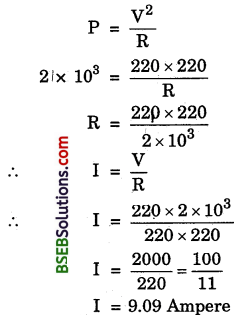At this stage, due to very high value of electric current, the oven will not function and would be damaged.

Question 3.
What precaution should be taken to avoid the overloading of domestic electric circuit ?
We can avoid overloading by not connecting too many appliances to a single socket.

### Bihar Board Class 10 Science Chapter 14 Sources of Energy Textbook Questions and Answers

Question 1.
Which of the following correctly describes the magnetic field near a long straight wire ?
(a) The field consists of straight lines perpendicular to the wire.
(b) The field consists of straight lines parallel to the wire.
(c) The field consists of radial lines’originating from the wire.
(d) The field consists of concentric circles centred at the wire.
(d) The field consists of concentric circles centred at the wire.

Question 2.
The phenomenon of electro-magnetic induction is :
(a) The process of charging a body.
(b) The process of generating magnetic field due to a current passing through a coil.
(c) Producing induced current in a coil due to relative motion between a magnet and the coil.
(d) The process of rotating a coil of an electric motor.
(c) Producing induced current in a coil due to relative motion between a magnet and the coil.

Question 3.
The device used for producing electric current is called a :
(a) generator
(b) galvanometer
(c) ammeter
(d) motor
(a) generatorQuestion 4.
The essential difference between an A.C. generator and a D.C. generator is that :
(a) A.C. generator has an electro-magnet while a D.C. generator has permanent magnet.
(b) D.C. generator will generate a higher voltage.
(c) A.C. generator will generate a higher voltage.
(d) A.C. generator has slip rings while the D.C. generator has a commutator.
(d) A.C. generator has slip rings while the D.C. generator has a commutator.

Question 5.
At the time of short-circuit, the current in the circuit :
(a) reduces substantially.
(b) does not change.
(c) increases heavily.
(d) vary continuously.
(c) increases heavily.

Question 6.
State whether the following statements are true or false :
(a) An electric motor converts mechanical energy into electrical energy.
(b) An electric generator works on the principle of electromagnetic induction.
(c) The field at the centre of a long circular coil carrying current will be parallel straight lines.
(d) A wire with green insulation is usually the live wire.
(a) False
(b) True
(c) True
(d) False

Question 7.
List three sources of magnetic field.
The three sources of magnetic field are as follows :
(i) A nature magnet has magnetic field around it.
(ii) A current-carrying straight or circular conductor has magnetic field around it.
(iii) A current-carrying solenoid has magnetic field around it.

Question 8.
How does a solenoid behave like a magnet ? Can you determine the north and south poles of a current-carrying solenoid with a help of a bar magnet ? Explain.
A coil of many circular turns of insulated copper wire wrapped closely in the shape of a cylinder is called a solenoid. One end of the solenoid behaves like the magnetic north pole, while the other end behaves as the south pole. The field lines inside the solenoid are in the form of parallel lines. This indicates that the magnetic field is the same at all points inside the solenoid. So, the field is uniform inside the solenoid.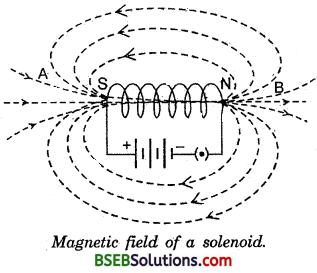Yes, we can determine the north and south poles of solenoid I with the help of a magnetic needle which is a magnet. On bringing the magnetic needle towards one end of the solenoid, if the north pole of the magnetic needle is attracted, then the end under consideration is the south pole and vice-versa.

Question 9.
When is the force experienced by a current- carrying conductor placed in a magnetic field largest ?
We know that the force experienced by a current-carrying conductor in a magnetic field is given as
F = BIL sin θ
B = magnetic field,
I = strength of current in the
1 conductor,
L = length of conductor,
F = force
θ = angle between direction of magnetic field and current carrying conductor.
So, the force is maximum if 0 = 90° as sin 90° = 1 is the maximum value of sin θ.

Question 10.
Think you are sitting in a chamber with your 1 back to one wall. An electron beam, moving horizontally from back wall towards the front wall is deflected by a strong magnetic field to your right side. What is the direction of magnetic field ?
The magnetic field will be vertically downwards.Question 11.
Draw a labelled diagram of an electric motor. Explain its principle and working. What is the function of a split ring in an electric motor ?
(i) Principle : When a current- carrying conductor is placed in a magnetic field, it experiences a force in the direction given by Fleming’s left hand rule.

Working : In an electric motor, a coil of wire having many turns is wrapped around a cylindrical axle. This rectangular coil is placed between the pole pieces of a magnet as shown in Fig.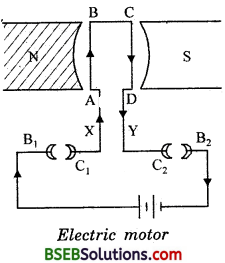When a current passes through the coil, entering at point X and leaving at point Y, the two arms AB and CD, which are perpendicular to the direction of the magnetic field, experience a force according to Fleming’s left hand rule. Since the direction of the current in the two arms is opposite to each other, the forces acting on them will also be opposite to each other. These forces push one arm (CD) up and the other arm (AB) down. As the coil is free to rotate about an axis, it will complete half rotation in anti-clockwise direction.

The split rings C1 and C2 also rotate with the coil. After half rotation, C1 comes in contact of brush B2 and C2 comes in contact of brush Blf hence the direction of current in the coil is reversed. The current of the coil is now from D to C and then from B to A. According to Fleming’s left hand rule, GD will move down and AB will move up. The coil* therefore, rotates half a turn more where the current in the coil is again reversed. In this way, a reversing process is repeated at each half turn giving rise to a continuous rotation.

(ii) The function of the commutator is to alter the direction of current in the coil after every half a rotation.

Question 12.
Name, some devices in which electric motors are used.
The name of devices are :
(a) cooler
(b) A.C.
(c) fan
(e) motor cycle, etc.

Question 13.
A coil of insulated copper wire is connected to a galvanometer. What will happen if a bar magnet is (i) pushed into the coil, (it) withdrawn from inside the coil, (iii) held stationary inside the coil ?
In this case, current will be induced in the coil which will be indicated by deflection in the needle of the galvanometer. For giyen cases, the explanation is given below :
(i) When a bar magnet with its north pole facing the coil is > moved towards the coil, the needle of galvanometer will move momentarily in one direction.
(ii) When the bar magnet is moved away from the coil, the needle of galvanometer will move momentarily but opposite direction to (i).
(iii) When the bar magne,t is stationary near the coil, no deflection in the needle would be observed.

Question 14.
Two circular coils A and B are placed close to each other. If the current in the coil A is changed, will some current be induced in the coil B ? Give reason.
Yes, we know that larger the current, stronger will be the magnetic field, i.e., no. of magnetic field lines passing through
a given area is more. As there is change in current in coil A, there is a decrease in the no. of magnetic field lines passing through coil B and, hence, due to change in the no. of magnetic field lines, there is an induced current in coil B.Question 15.
State the rule to determine the direction of a (i) magnetic field produced around a straight conductor carrying current,
(ii) force experienced by a current-carrying straight conductor placed in a magnetic field which is perpendicular to it, and
(iii) current induced in a coil due to its rotation in a magnetic field.
Following rules are taken under consideration for given cases :

Case I : Direction of magnetic field at a point near current-carrying conductor is given by the right hand thumb rule.
If a current – carrying conductor is 7 to be held in the right hand such that the thumb points in the direction of the current, then the curbed fingers of the hand indicate the direction of magnetic field.

Case II : The direction of force on current-carrying conductor is given by Fleming’s left hand rule, which is stated as below : “Stretch the left hand such that the thumb, first finger and the central finger are mutually perpendicular to each other. If the first finger points in the direction of magnetic field and central finger points in the direction of current, then the thumb will point in the direction of motion (or force).”

Case III : The direction of induced current in a coil is given by Fleming’s right hand rule, which is stated as below :
“Stretch the right hand such that the first finger, the central finger and the thumb are mutually perpendicular to each other. If the first finger points along the direction of the field and the thumb indicates the direction of motion of the conductor, then the direction of induced current is given by the direction of the central finger.”

Question 16.
Explain the underlying principle and working of an electric generator by drawing a labelled diagram. What is the function of brushes ?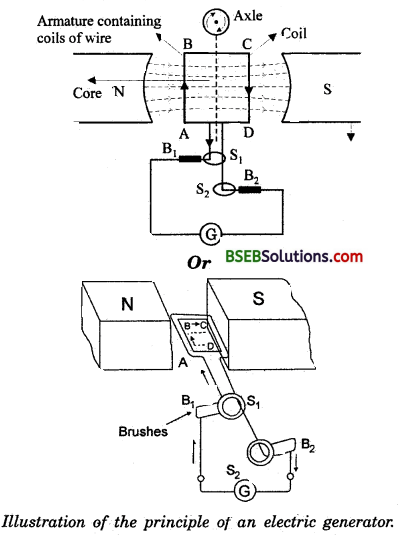Principle of electric generator :
Electric generator is based on the phenomenon of elctromagnetic induction, i.e., a changing magnetic field in a conductor induces an electric current in the conductor. Direction of the induced current is given by Fleming’s right hand rule. In an electric generator, the magnetic field in the conductor is changed by moving the conductor in the magnetic field of the magnet.

Construction : It consists of a rotating armature ABCD containing coils of wire, pole pieces, brushes and a commutator. The two ends of the. armature ABCD are connected to two metallic rings Si and S2 . The two brushes Bx and B2 , which are connected to a galvanometer G, are in contact with the rings Sx and S2 respectively.

Working : In an electric generator, mechanical work is done to turn the armature. As the coil rotates in the magnetic field, the two rings also rotate with the coil but the same brushes keep contact with the rotating rings. During the rotation of coil, its arm AB moves up and arm CD moves down cutting the magnetic lines of feree, so induced current generates in the arms AB and CD in the direction A to B and C to D according to Fleming’s right hand rule. Hence we get current flowing in the direction ABCD. As there are a number of turns in the coil, the current generated in each turn adds up to give a large current through the coil. This current will flow in the external circuit in the direction as shown in Fig.

After half a rotation, now CD starts moving up and AB moves down. As a result, the direction of induced current in both the arms AB and CD changes. This gives rise to induced current in the direction DCBA and the current in the external circuit will flow in the opposite direction.

Thus, after every half a rotation, the polarity of the current in the arms AB and CD changes. Such a current which changes direction after equal intervals of time is called an alternating current. Since this electric generator is producing alternating current, it is also called A. G. generator. Function of the brushes : The carbon brushes are fixed while slip rings rotate along with the armature. These brushes are connected to the load through which the output is obtained.Question 17.
When does an electric short circuit occur ?
When the live and neutral wires come in direct contact with each other, a very large current passes through the circuit. Then it is said that “short circuiting” has occurred.

Question 18.
What is the function of an earth wire ? Why is it necessary to earth metallic appliances ?
Connecting the metal case of an electric appliance to the earth by means of a metal wire is called earthing. It is used as a safety measure specially for those appliances which have a metallic body. The metallic body is earthened which provides a low resistance conducting path for the current. Thus, if there is any leakage of the current, the user doesn’t get any severe shock.

### Bihar Board Class 10 Science Chapter 14 Sources of Energy Textbook Activities

Activity 13.1 (Page 223)

• Take a straight thick copper wire and place it between the points X and Y in an electric circuit as shown in Fig. 13.1 of your book.
• Place a small compass near to this copper wire. See the position of its needle.
• Pass the current through the circuit by inserting the key into the plug.

Question 1.
Observe the change in the position of the compass needle.
Compass needle is deflected on passing an electric current through a metallic conductor.

Activity 13.2 (Page 224)

• Fix a sheet of white paper on a drawing board using some adhesive material.
• Place a bar magnet in the centre of it.
• Sprinkle some iron filings uniformly around the bar magnet (Fig. 13.2 of your book). A salt-sprinkler may be used for this purpose.
• Now tap the board gently.

Questions Based on the Activity

Question 1.
What do you observe ?
Iron filings near the bar magnet align themselves along the field lines.

Question 2.
Why do the iron filings arrange in such a pattern ?
The iron filings are arranged in such a pattern because the magnet exerts its influence in the region surrounding it. Therefore, the iron filings experience a force and get arranged.

Question 3.
What does this pattern demonstrate ?
This pattern demonstrates that the lines along which the iron filings align themselves are the magnetic field lines.Question 4.
What do the crowded no. of iron filings at the end of magnet indicate ?
This indicates that the magnetic field strength of a magnet is maximum at the ends of a magnet.

Activity 13.3 (Page 224)

Questions Based on the Activity

Question 1.
How can you detect the direction of magnetic field lines by a magnet ?
With the help of a magnetic needle, we can detect the direction of magnetic field around a given magnet at a point.

Question 2.
What is the direction of magnetic field lines of a magnet ?
The magnetic field fines originate from the north pole and terminate at the south end of the magnet.

Question 3.
Where are the magnetic field lines more denser ?
Magnetic field fines are more denser at the poles of a magnet.

Activity 13.4 (Page 226)

Questions Based on the Activity

Question 1.
What happens when a current* carrying conductor is placed over a compass needle ?
There is deflection of the north pole of the needle if the direction of the current is from north to south. [SNOW Rule]

Question 2.
What will happen when the direction of current is changed in conductor ?
The direction of deflection of the magnetic needle is also changed.

Question 3.
What will happen when the direction of current is reversed in conductor kept near a compass needle?
The direction of deflection of needle is also reversed.

Activity 13.5 (Page 226)

Questions Based on the Activity

Question 1.
What is the nature of magnetic field lines around a current-carrying straight conductor ?
These magnetic field lines are in form of concentric circles having their centre at the conductor.

Question 2.
What do these concentric circles represt nt ?
They represent the magnetic field lines.

Question 3.
How can the direction of the magnetic field be found ?
Place a compass at a point over a circle. The direction of the north pole of the compass needle would give the direction of the magnetic field lines at that point.Activity 13.6 (Page 229)

Questions Based on the Activity

Question 1.
Is there a magnetic field around a current¬carrying circular conductor ?
Yes, there is a magnetic field around a current-carrying circular conductor.

Question 2.
What is the nature of magnetic field lines at two N points opposite to each other on circular current¬carrying conductor ?
The direction of magnetic field lines is opposite on both the points, but at both points, there will be circular and concentric field lines.

Question 3.
Where will the value of magnetic field be maximum due to current-carrying circular conductor ?
At the centre of circular current-carrying conductor, the value of the magnetic field is maximum.

Activity 13.7 (Page 230)

Questions Based on the Activity

Question 1.
What happens when a current-carrying conductor is placed in a magnetic field ? ‘
A force acts on a current-carrying conductor when it is placed in a magnetic field.

Question 2.
Only name the law which is used to find the direction of force on a conductor carrying current when placed in a magnetic field ?
Fleming’s left hand rule.

Question 3.
On which factor strength of force depends when a conductor carrying current is placed in a magnetic field?
The factors are :
(a) magnitude of the magnetic field.
(b) length of the conductor.
(c) strength of current through conductor.

Question 4.
What will happen when the direction of current through current-carrying conductor is reversed ?
The direction of force on current-carrying conductor is reversed.

Activity 13.8 (Page 233)

Take a coil of wire AB having a large number of turns.

Connect the ends of the coil to a galvano- meter as shown in Fig. 13.16 of your book.

Take a strong bar magnet and move its north pole towards the end B of the coil. Do you find any change in the galvanometer needle ?

There is a momentary deflexion in the needle of the galvanometer, say to the right. This indicates the presence of a current in the coil AB. The deflection becomes zero the moment the motion of the magnet stops.

Now withdraw the north pole of the magnet away from the coil. Now the galvanometer is deflected toward the left, showing that the current is now set up in the direction opposite to the first.Place the magnet stationary at a point near to the coil, keeping its north pole towards the end B of the coil. We see that the galvanometer needle deflects toward the right when the coil is moved towards the north pole of the magnet. Similarly the needle moves toward left when the coil is moved away.

When the coil is kept stationary with respect to the magnet, the deflection of the galvanometer drops to zero.

Question 1.
What do you conclude from this activity ?
If the motion of a magnet with respect to the coil produces an induced potential difference, which sets up an induced electric current in the circuit.

Activity 13.9 (Page 235)

Questions Based on the Activity

Question 1.
What happens when a current-carrying coil is brought nearer to the another coil ?
Induced current is developed in the second coil.

Question 2.
What is the value of induced current if there is steady current in coil I ? ’
The galvanometer will show no deflection and the reading of galvanometer will be zero.

Question 3.
What are the names of current-carrying coil and another coil connected to galvanometer ?
The current-carrying coil is called primary coil and the other is called secondary .coil.

Question 4.
Which will produce magnetic field either primary or secondary coil ?
Primary coil will produce magnetic field due to which induced current is developed in the secondary coil.

Question 5.
Write the factors on which strength of induced current depends in secondary coil ?
Factors are :
(а) Strength of current in primaxy coil.
(b) Number of turns in primary coil.

Question 1.
Explain different ways to induce current in a coil. Ans. Following would be the different ways to induce current in a coil :
(а) Either coil or a magnet should be in motion relative to each other, then there vrill be an induced current in the coil.
(b) If there is relative motion between a current-carrying coil and a coil without current, then there will be an induced current in the second coil.

### Bihar Board Class 10 Science Chapter 14 Sources of Energy NCERT Exemplar Problems

Question 1.
A magnetic compass needle is placed in the plane of paper near point A as shown in the given figure. In which plane should a straight current carrying conductor be placed so that it passes through A and there is no change in the deflection of the compass? Under what condition is the deflection maximum and why? .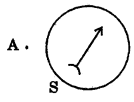In the plane of the paper itself. The axis of the compass is vertical and the field due to the conductor is also vertical. It could result in a dip of compass needle which is not possible in this case (dips result only if axis of compass is horizontal). The deflection is maximum when the conductor through A is perpendicular to the plane of paper and the field due to it is maximum in the plane of the paper.Question 2.
Under what conditions permanent electromagnet is obtained if a current carrying solenoid is used? Support your answer with the help of a labelled circuit diagram.
(i) The current through the solenoid should be direct current.
(ii) The rod inside is made’of a magnetic material such as steel.Question 3.
AB is a current carrying conductor in the plane of the paper as shown in the given figure. What are the directions of magnetic fields produced by it at points P and Q1 > Q2 where will the strength of the magnetic field be larger?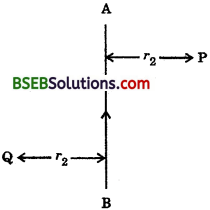The magnetic field will be larger into the plane of paper at P and out of it at Q. The strength of the magnetic field is larger at the point located closer i.e., at Q.

Question 4.
A magnetic compass shows a deflection when placed near a current carrying wire. How will deflection of the compass get affected if the current in the wire is increased? Support your answer with a reason.
The deflection increases. It is because the strength of magnetic field is directly proportional to the magnitude of current passing through the straight conductor.

Question 5.
It is established that an electric current through a metallic conductor produces a magnetic field around it. Is there a similar magnetic field produced around a thin beam of moving (£) alpha particles, (ii) neutrons? Justify your answer.
(i) Yes. Alpha particles being positively charged constitute a current in the direction of motion.
(ii) No. The neutrons being electrically neutral constitute no current.Question 6.
What does the direction of thumb indicate in the right hand thumb rule ? In what way this rule is different from Fleming’s left hand rule?
The thumb indicates the direction of current in the straight conductor held by Curled fingers, whereas the Fleming’s left hand rule gives the direction of force experienced by current carrying conductor placed in an external magnetic field.

Question 7.
Meena draws magnetic field lines of field close to the axis of a current carrying circular loop. As she moves away from the centre of the circular loop she observes that the lines keep on diverging. How will you explain her observation.
Strength of the magnetic field falls as distance increases. This is indicated by the decrease in degree of closeness of the lines of field. .

Question 8.
What does the divergence of magnetic Held lines near the ends of a current carrying straight solenoid indicate?
The divergence, that is, the falling degree of closeness of magnetic field lines indicates the fall in strength of magnetic field near and beyond the ends of the solenoid.

Question 9.
Name four appliances wherein an electric motor, a rotating device that converts electrical energy to mechanical energy, is used as an important component. In what respect motors are different from generators?
Electric fans mixers, washing machines and computer drives. Motors convert electrical energy into mechanical energy whereas generators convert mechanical energy into electrical energy.

Question 10.
What is the role of the two conducting stationary brushes in a simple electric motor?
The brushes are connected to the battery and touch the outer side of two halves of the split ring whose inner sides are insulated and attached to the axle.

Question 11.
What is the difference between a direct current and an alternating current? How many times does AC used in India change direction in one second?
Direct current always flows in one direction but the alternating current reverses its direction periodically. The frequency of AC in India is 50 Hz and in each cycle it alters direction twice. Therefore AC changes direction 2 x 50 = 100 times in one second.Question 12.
What is the role of fuse, used in series with any electrical appliance? Why should a fuse with defined rating not be replaced by one with a larger rating?
Fuse is used for protecting appliances due to short- circuiting or overloading. The fuse is rated for a certain maximum current and blows off when a current more than the rated value flows through it. If a fuse is replaced by one with larger ratings, the appliances may get damaged while the protecting fuse does not burn off. This practice of using fuse of improper rating should always be avoided.

Question 13.
In wait way these simple electric motors are diffferent from commercial motors?
Commercial motors use an electromagnet in place of a permanent magnet, a large number of turns of conducting wire in the current carrying coil and a Soft iron core on which the coil is wound.

uestion 14.
What changes must be made in the arrangement to convert it to a DC generator?
To convert an AC generator into a DC generator a split ring type commutator must be used in place of slip ring type commutator.

Question 15.
Why is it that a burnt out fuse should be replaced s by another fuse of identical rating?
It is because we should use the fuse wire whose capacity is slightly more than the maximum current that can flow in it. ^ Different live wires are used to supply electricity to different appliances and so fuse should be used accordingly.1 Long Answer Type Questions

Question 16.
Why does a magnetic compass needle pointing North and South in the absence of a nearby magnet get ‘ deflected when a bar magnet or a current carrying loop is brought near it. Describe some salient features of magnetic lines of field concept.
Current carrying loops behave like bar magnets and i both have their associated lines of field. This modifies the already existing earth’s magnetic field and a deflection results. Magnetic field has both direction and magnitude. Magnetic field lines emerge from N-pole and enter S-pole. The magnetic field strength ” is represented diagrammatically by the degree of closeness of
the field lines. Field fines cannot cross each other as two values 1 of net field at a single point cannot exist. Only one value, a unique net value, can exist. If in a given region, fines of field are shown to be parallel and equispaced, the field is understood to be uniform.Question 17.
With the help of a labelled circuit diagram illustrate the pattern of field lines of the magnetic field around a current carrying straight long conducting wire. How is the right hand thumb rule useful to find direction of magnetic field associated with a current carrying conductor?
Right hand thumb rule states that if a current carrying straight conductor is supposedly held in the right hand with the thumb pointing towards the direction of current, then the fingers will wrap around the conductor in the direction of the field lines of the magnetic field.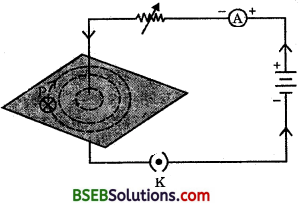Question 18.
Explain with the’ help of a labelled diagram the distribution of magnetic field due to a current through a circular loop. Why is it that if a current carrying coil has n turns the field produced at any point is n times as large as that produced by a single turn?